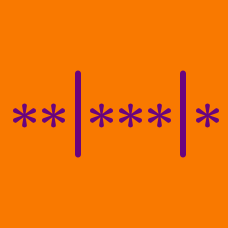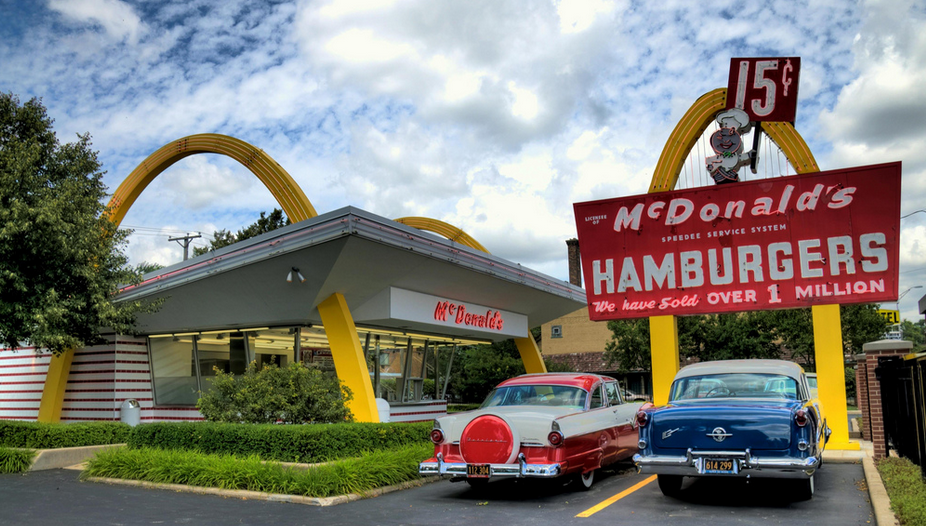Probability

# Distribution into Bins: Level 3 ChallengesOnce Calvin and his friends were very hungry and they decided to go to the McDonalds to satisfy their craving.

In the McD menu, there were 4 burgers mentioned: McVeggie, McChicken, Chicken Maharaja - Mac, and Filet-o-Fish.

If Calvin ordered 10 burgers in total, in how many ways can Calvin buy the burgers? Note that the order does not matter.

How many three digit numbers are there such that the sum of the digits in each number is 10.

How many (positive) seven digit integers are there that have a digit sum equal to $10$ and that are formed using only the digits $1$, $2$ and $3$?

There are five distinct balls and three distinct urns. Each urn can contain all five balls. In how many ways can the balls be placed in the urns such that no urn remains empty?

How many ordered pairs of non-negative integers $(M, N)$ are there which satisfy the inequality $M + N \leq 25$?

×

Problem Loading...

Note Loading...

Set Loading...